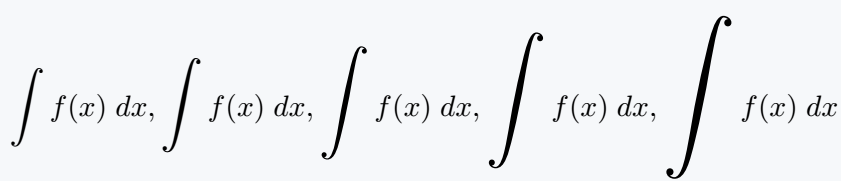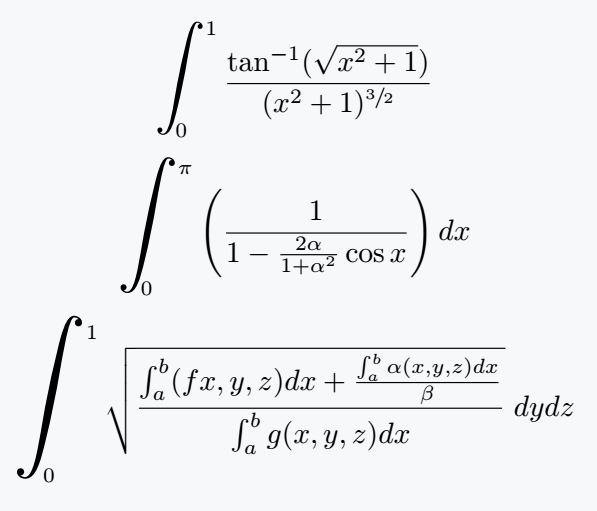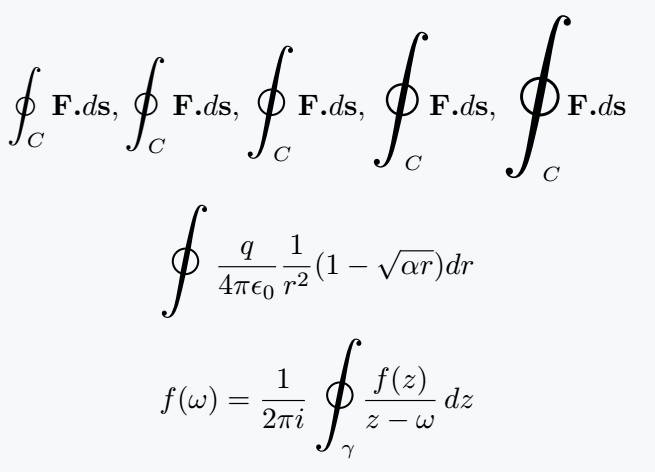# How to write a big integral in LaTeX?

To make a document beautiful, every symbol and expression of latex must match. For this reason, Big Symbol will be needed from time to time.

The popular package for big integral symbol is bigints, in which different commands are defined in sequence.

\documentclass{article}
\usepackage{bigints}
\begin{document}
$\bigintssss f(x)\;dx,\bigintsss f(x)\;dx,\bigintss f(x)\;dx,\bigints f(x)\;dx,\bigint f(x)\;dx$
\end{document}

Output :## Big integral symbol with limits

Since the size of the integral symbol is large. So, there is no need to use the \limits command. So the limit has been used with the direct big integral symbol

\documentclass{article}
\usepackage{xfrac,bigints}
\begin{document}
$\bigintss_0^1\frac{\tan^{-1}(\sqrt{x^2+1})}{(x^2+1)^{\sfrac{3}{2}}}$
$\bigints_0^\pi\left(\frac{1}{1-\frac{2\alpha}{1+\alpha^2}\cos x}\right)dx$
$\bigint_0^1 \sqrt{\frac{\int_a^b(fx,y,z)dx+\frac{\int_a^b \alpha(x,y,z)dx}{\beta}}{\int_a^b g(x,y,z)dx}}\;dydz$
\end{document}

Output :## Close integral symbol in LaTeX

In the same way, the command is defined in chronological order for Big Close integral. Which is shown in the following code.

\documentclass{article}
\usepackage{bigints}
\begin{document}
$\bigointssss_C\textbf{F.}d\textbf{s},\bigointsss_C\textbf{F.}d\textbf{s},\bigointss_C\textbf{F.}d\textbf{s},\bigoints_C\textbf{F.}d\textbf{s},\bigoint_C\textbf{F.}d\textbf{s}$
$\bigointss\frac{q}{4\pi\epsilon_0}\frac{1}{r^2}(1-\sqrt{\alpha r})dr$
$f(\omega)=\frac{1}{2\pi i}\bigointss_\gamma\frac{f(z)}{z-\omega}\,dz$
\end{document}

Output :#### Md Jidan Mondal

LaTeX expert with over 10 years of experience in document preparation and typesetting. Specializes in creating professional documents, reports, and presentations using LaTeX.